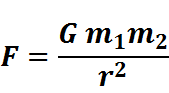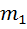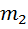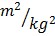# Fine-Tuning of the Universe (part 2 of 8): Constants & Initial Conditions

Rating:
Font size:

### Description: Simple explanation of what is meant by the fine-tuning of the constants of nature and the initial conditions of the universe.

• By Imam Mufti (© 2014 IslamReligion.com)
• Published on 07 Apr 2014
• Printed: 168
• Viewed: 41,670 (daily average: 12)
• Rating: 5.0 out of 5
• Rated by: 12
• Emailed: 1
• Commented on: 0
Poor Best

### 2.  Fine-Tuning of the ConstantsWhat is a constant? What is meant are the constants of physics.  When the laws of nature are expressed as mathematical equations, like the force of gravity, the electromagnetic force, and the subatomic ‘weak’ force, you find certain symbols in them that stand for numbers that don’t change.  These unchanging numbers are called ‘constants’ that occur in the laws of physics.

The laws of nature do not determine the value of these constants.  There could be a universe governed by the same laws, but with different values of these constants.  Therefore, the actual values of these constants are not determined by the laws of nature.  Depending on the values of those constants, a universe governed by the same laws of nature will look very different.

There are at least 20 independent constants and factors that are fine-tuned to a high degree of precision for life to be possible in the universe.  It is estimated that every year roughly another number is added to the list.

G: Example of a Finely-Tuned Constant

An example of a constant is the Gravitational constant – designated by G – which determines the strength of gravity via Newton’s Law of Gravity.F is the force between two massesandthat are at a distance r apart.  The actual value of G is 6.67 x 10-11  N.  Increase or decrease G and the force of gravity will correspondingly increase or decrease.

If one increased the strength of gravity by one part in 1034, even single-celled organisms would be crushed and only planets less than around 100 feet in diameter would sustain life with our brain-size.  Such planets, however, could not sustain an ecosystem to support life of our level of intelligence. In fact even a basic ecosystem can barely be possible on such a place.

If fact, if G were increased by a mere 64-fold, the gravitational force of the surface of any planet that could retain an atmosphere would be at least 4 times as large.  A 400-fold increase in G would result in any such planet having a surface force at least 10 times as large.  Such a planet would be far less ideal than earth for humans.  On the other hand, a small decrease in G would negatively affect the planet’s hydrologic cycle, this also making any habitable planet less ideal.

### 3.  Fine-Tuning of the Initial Conditions of the Universe

In addition to the constants, there are certain arbitrary quantities that are just put in as initial conditions on which the laws of nature operate.  Because these quantities are arbitrary, they are also not determined by the laws of nature.

I will first give a simple example to explain what it means.  When I throw a ball, I throw it at a certain angle and with certain speed.  The angle and speed are the ‘initial conditions.’ After I throw it, the ball follows a certain course, and where the ball lands will depend on the ‘initial conditions.’ The course taken by the ball is calculated by using the law of gravity, which is one of the laws of physics.

Now, take an example of entropy (thermodynamic disorder) in early universe.  It is an ‘initial condition’ in the Big Bang model similar to the speed and angle for the ball in the example above.  Just like the example of the ball, after the Big Bang, the laws of physics take over and determine how the universe will develop from there on.  If the initial entropy (an initial condition) of the universe had been different, the laws would predict a very different universe.

Here is the amazing part.  Scientists have discovered that that these constants and initial conditions must fall into an extremely narrow range of values for the universe to exist.  This is what is meant by ‘the universe has been fine-tuned for life.’

Footnotes:

 Spitzer, Robert.  2010.  New Proofs for the Existence of God: Contributions of Contemporary Physics and Philosophy.  Grand Rapids/Cambridge: Wm.B.  Eerdmans Publishing Co.  50-56.

 The calculations were done and presented by Dr.  Robin Collins, Professor of Philosophy and Chair of the Department of Philosophy at Messiah College, at Pepperdine University lecture titled ‘Is [it] True?’ hosted by the Veritas Forum on Feb 18, 2013.

Poor Best

View all parts together

• (Not shown to the public)

• Your comment will be reviewed and should be published within 24 hours.

Fields marked with an asterisk (*) are required.

## Other Videos in the Same Category

Daily
Total

#### List Contents

This list is currently empty.
All by date

#### Most Popular

Highest rated
Most emailed
Most printed
Most commented on